# Pythagorean theorem - examples - page 7

1. PitPit has shape of a truncated pyramid with rectangular bases and is 0.8 m deep. The length and width of the pit is the top 3 × 1.5 m bottom 1 m × 0.5 m. To paint one square meter of pit we use 0.6 l of green colour. How many liters of paint is needed when w
2. Elevation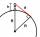What must be the elevation of an observer in order that he may be able to see an object on the earth 536 km away? Assume the earth to be a smooth sphere with radius 6378.1 km.
3. Triangle and its heightsCalculate the length of the sides of the triangle ABC, if va=5 cm, vb=7 cm and side b is 5 cm shorter than side a.
4. Euclid2In right triangle ABC with right angle at C is given side a=27 and height v=12. Calculate the perimeter of the triangle.
5. Sea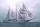How far can you see from the ship's mast, whose peak is at 14 meters above sea level? (Earth's radius is 6370 km).
6. CircleWrite the equation of a circle that passes through the point [0,6] and touch the X-axis point [5,0]: ?
7. Trapezoidtrapezoid ABCD a = 35 m, b=28 m c = 11 m and d = 14 m. How to calculate its area?
8. MO circlesJuro built the ABCD square with a 12 cm side. In this square, he scattered a quarter circle that had a center at point B passing through point A and a semicircle l that had a center at the center of the BC side and passed point B. He would still build a ci
9. Triangle KLBIt is given equilateral triangle ABC. From point L which is the midpoint of the side BC of the triangle it is drwn perpendicular to the side AB. Intersection of perpendicular and the side AB is point K. How many % of the area of the triangle ABC is area o
10. BoxCalculate the angle between box base 9 x 14 and body diagonal length 18.
11. Circumscribing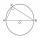Determine the radius of the circumscribed circle to the right triangle with legs 9 cm and 6 cm.
12. Glass mosaicHow many dm2 glass is nessesary to produc 97 slides of a regular 6-gon, whose side has length 21 cm? Assume that cutting glass waste is 10%.
13. Distance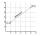Calculate distance between two points X[18; 19] and W[20; 3].
14. Tank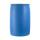In the middle of a cylindrical tank with a bottom diameter 251 cm is standing rod which is 13 cm above the water surface. If we bank rod its end reach surface of the water just by the tank wall. How deep is the tank?
15. Inscribed rectangle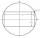The circle area is 216. Determine the area of inscribed rectangle with one side 5 long.
16. Triangular prismBase of perpendicular triangular prism is a right triangle with leg length 5 cm. Content area of the largest side wall of its surface is 130 cm² and the height of the body is 10 cm. Calculate its volume.
17. Rectangle diagonalsIt is given rectangle with area 24 cm2 a circumference 20 cm. The length of one side is 2 cm larger than length of second side. Calculate the length of the diagonal. Length and width are yet expressed in natural numbers.
18. Axial sectionAxial section of the cylinder has a diagonal 40 cm. The size of the shell and the base surface are in the ratio 3:2. Calculate the volume and surface area of this cylinder.
19. CirclesArea of circle inscribed in a square is 14. What is the area of a circle circumscribed around a square?
20. Isosceles IIIThe base of the isosceles triangle is 17 cm area 416 cm2. Calculate the perimeter of this triangle.

Do you have an interesting mathematical example that you can't solve it? Enter it, and we can try to solve it.

To this e-mail address, we will reply solution; solved examples are also published here. Please enter e-mail correctly and check whether you don't have a full mailbox.

Pythagorean theorem is the base for the right triangle calculator.